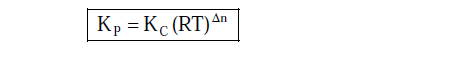Welcome to Chem Zipper.com......: Relation between Kp and Kc and Law of Mass of action: Equilibrium constants:

## Tuesday, August 29, 2023

### Relation between Kp and Kc and Law of Mass of action: Equilibrium constants:

LAW OF MASS ACTION: Law of mass action is applicable for only reversible chemical reactions and it is an imperial law.

The law state that “ At a fixed temperature the rate of a chemical reaction is directly proportional to the product of reactive mass of reactants raised to the their respective Stoichiometric coefficients ” The law of mass action is by Guldberg and Waage.

(1) Equilibrium Constants in term of concentration ( KC):
At the constant temperature, let us consider the following reversible reactionAccording to law of mass action - The rate of forward reaction
The rate of reverse reaction-
Where Kf and Kb  is the rate constant of the forward reaction and backward reaction respectively
We know at equilibrium, the two rates of forward as well as backward are equal. ie
Rate of reaction = Rate of forward reaction – Rate of backward reaction = 0
Kc=Kf/Kb
Unit of Kc= (Conc)ng
(2) Equilibrium Constants in term of Pressure( Kp):
From law of mass action- rate forward reaction is directly proportional  to product of active mass of reactants and rate backward reaction is directly proportional  to product of active mass of products.

### T=Temperature in kelvin

= total number of moles of gaseous products -total number of moles of gaseous reactantsIllustrated examples: